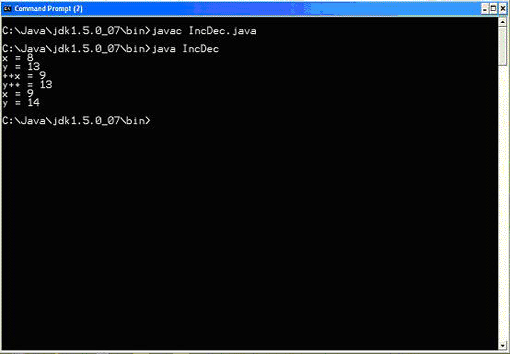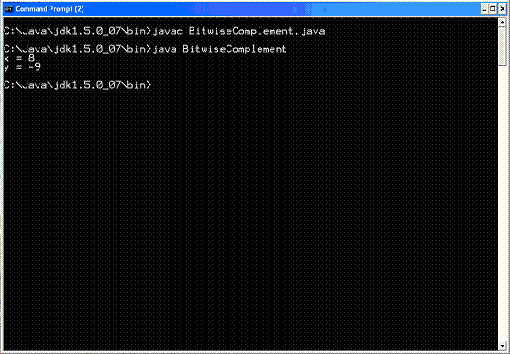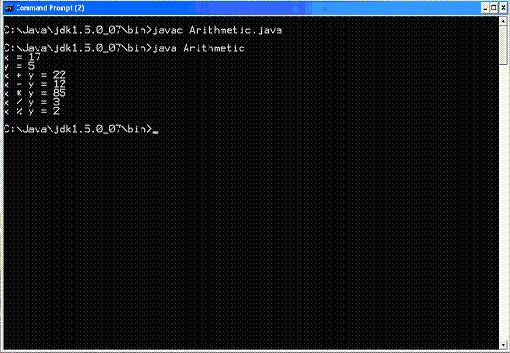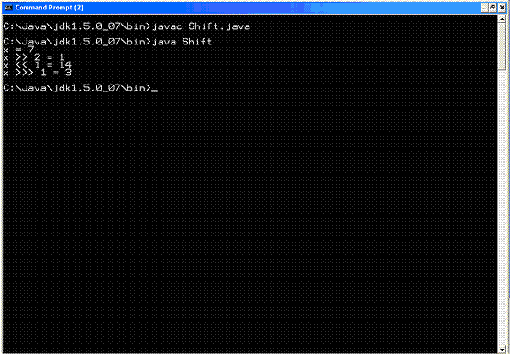## Core Java

Java Evolution
Overview of Java Language
Constant, Variable and Datatype
Operator and Expression
Decision making, Branching and Looping
Class Object and Method
Array and String
Inheritance
Package
Exception Handling
Applet
Managing Input/Output Files in Java
Examples of Core Java

# Operator and Expression

### Integer Operators

 There are three types of operations that can be performed on integers: 1. unary, 2. binary, 3. And relational. Unary operators act on only single integer numbers, Binary operators act on pairs of integer numbers. Both unary and binary integer operators typically return integer results. Relational operators, act on two integer numbers but return a Boolean result rather than an integer. Unary and binary integer operators typically return an int type. For all operations involving the types byte, short, and int, the result is always an int. The only exception to this rule is when one of the operands is a long, in which case the result of the operation is also of type long.

Unary Integer Operators
Unary integer operators act on a single integer. Lists of the unary integer operators.

The unary integer operators.

 Description Operator Increment ++ Decrement -- Negation - Bitwise complement ~

The increment and decrement operators (++ and --) increase and decrease integer ariables by 1.

These operators can be used in either prefix or postfix form. A prefix operator takes effect before the evaluation of the expression it is in; a postfix operator takes effect after the expression has been evaluated.

Prefix unary operators are placed immediately before the variable; postfix unary operators are placed immediately following the variable. Following are examples of each type of operator:
y = ++x;
z = x--;

In the first example, x is prefix incremented, which means that it is incremented before being assigned to y.
In the second example, x is postfix decremented, which means that it is decremented after being assigned to z. Here z is assigned the value of x before x is decremented.
The IncDec program, which uses both types of operators.

The IncDec class.

class IncDec
{
public static void main (String args[])
{
int x = 8, y = 13;
System.out.println("x = " + x);
System.out.println("y = " + y);
System.out.println("++x = " + ++x);
System.out.println("y++ = " + y++);
System.out.println("x = " + x);
System.out.println("y = " + y);
}

}

The IncDec program produces the following results:

x = 8
y = 13
++x = 9
y++ = 13
x = 9
y = 14The negation unary integer operator (-) is used to change the sign of an integer value.
x = 8;
y = -x;

In this example, x is assigned the literal value 8 and then is negated and assigned to y.
The resulting value of y is -8.

 The Negation class. class Negation { public static void main (String args[]) { int x = 8; System.out.println("x = " + x); int y = -x; System.out.println("y = " + y); } } The bitwise complement operator (~), which performs a bitwise negation of an integer value. Bitwise negation means that each bit in the number is toggled. In other words, all the binary 0s become 1s and all the binary 1s become 0s. Example: x = 8; y = ~x;
In this example, x is assigned the literal value 8 again, but it is bitwise complemented before being assigned to y.

Well, without getting into the details of how integers are stored in memory, it means that all the bits of the variable x are flipped, yielding a decimal result of -9. This result has to do with the fact that negative numbers are stored in memory using a method known as two's complement.

NOTE: Integer numbers are stored in memory as a series of binary bits that can each have a value of 0 or 1. A number is considered negative if the highest-order bit in the number is set to 1. Because a bitwise complement flips all the bits in a number--including the high-order bit--the sign of a number is reversed.
 The Bitwise Complement class. class BitwiseComplement { public static void main (String args[]) { int x = 8; System.out.println("x = " + x); int y = ~x; System.out.println("y = " + y); } }Binary Integer Operators
Binary integer operators act on pairs of integers. Lists of the binary integer operators.

The binary integer operators.

 Description Operator Addition + Subtraction - Multiplication * Division / Modulus % Bitwise AND & Bitwise OR | Bitwise XOR ^ Left-shift << Right-shift >> Zero-fill-right-shift >>>

The Arithmetic program, which shows how the basic binary integer arithmetic operators work.
 The Arithmetic class. class Arithmetic { public static void main (String args[]) { int x = 17, y = 5; System.out.println("x = " + x); System.out.println("y = " + y); System.out.println("x + y = " + (x + y)); System.out.println("x - y = " + (x - y)); System.out.println("x * y = " + (x * y)); System.out.println("x / y = " + (x / y)); System.out.println("x % y = " + (x % y)); } } The results of running the Arithmetic program follow: x = 17 y = 5 x + y = 22 x - y = 12 x * y = 85 x / y = 3 x % y = 2The bitwise AND, OR, and XOR operators (&, |, and ^) all act on the individual bits of an integer. These operators are sometimes useful when an integer is being used as a bit field.

The Bitwise class.

class Bitwise
{
public static void main (String args[])
{
int x = 5, y = 6;
System.out.println("x = " + x);
System.out.println("y = " + y);
System.out.println("x & y = " + (x & y));
System.out.println("x | y = " + (x | y));
System.out.println("x ^ y = " + (x ^ y));
}

}

The output of running Bitwise follows:

x = 5
y = 6
x & y = 4
x | y = 7
x ^ y = 3

In Bitwise, the variables x and y are set to 5 and 6, which correspond to the binary numbers 0101 and 0110.

The bitwise AND operation compares each bit of each number to see whether they are the same. It then sets the resulting bit to 1 if both bits being compared are 1; it sets the resulting bit to 0 otherwise. The result of the bitwise AND operation on these two numbers is 0100 in binary, or decimal 4.

The bitwise OR operator sets the resulting bit to 1 if either of the bits being compared is 1. For these numbers, the result is 0111 binary, or 7 decimal.

Finally, the bitwise XOR operator sets resulting bits to 1 if exactly one of the bits being compared is 1 and 0 otherwise. For these numbers, the result is 0011 binary, or 3 decimal.
The left-shift, right-shift, and zero-fill-right-shift operators (<<, >>, and >>>) shift the individual bits of an integer by a specified integer amount.

Following are some examples of how these operators are used:

x << 3;
y >> 7;
z >>> 2;

In the first example, the individual bits of the integer variable x are shifted to the left three places.
In the second example, the bits of y are shifted to the right seven places.
Finally, the third example shows z being shifted to the right two places, with zeros shifted into the two leftmost places.
 The Shift class. class Shift { public static void main (String args[]) { int x = 7; System.out.println("x = " + x); System.out.println("x >> 2 = " + (x >> 2)); System.out.println("x << 1 = " + (x << 1)); System.out.println("x >>> 1 = " + (x >>> 1)); } } The output of Shift follows: x = 7 x >> 2 = 1 x << 1 = 14 x >>> 1 = 3The number being shifted in this case is the decimal 7, which is represented in binary as 0111. The first right-shift operation shifts the bits two places to the right, resulting in the binary number 0001, or decimal 1. The next operation, a left-shift, shifts the bits one place to the left, resulting in the binary number 1110, or decimal 14. The last operation is a zero-fill-right-shift, which shifts the bits one place to the right, resulting in the binary number 0011, or decimal 3.